# VBScript Loops: Do While, Do Until, While, For Each (Example)

## Why Loops are used in VBScript?

Loops are used to execute the same block of code again and again. There are a number of VBScript looping statements. Some of the VBScript looping statements are Do While, Do Until, For…..Next and so on. So, how will you decide which looping statement to use for your need?

While executing the loops, you might or might not be aware of the number of times you want to execute a set of instructions. For example, you want to calculate the sum of two numbers ten times. In this case, you already know you want to repeat a block of code 10 times. Suppose, you want to calculate the sum of two numbers until a particular variable becomes true. Here, you do not know how many times the loop is to be executed. So based on whether you know or do not know the number of executions, you can use different types of looping statements.

For…Next looping statements are used if you want to repeat a block of code a finite number of times, that if you know the number of times if you want to repeat the block of code.

Do While and Do Until looping statements are used when you want to repeat a block of code until a condition becomes true or false, that is when you are not aware of the number of times you want to execute the block of code.

In this tutorial, you will learn-

Let’s take a look at these looping statements one by one.

## VBScript Do While Loop

If you do not know the number of times you need to execute a block of code, then you will be using Do While loops. For example, you want to output the message “Welcome” while the value of the variable x is less than 5. In such case, Do While loop will be used.

```<script type="text/vbscript">
Dim x
x=1
Do While x<5
document.write("Welcome.")
x=x+1
Loop
</script>	```

If you try executing the code, you will get the output like this: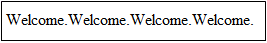The block gets executed four times (when x=1,2,3 and 4) and the loop ends when the value of x becomes 5. If you forget the statement x=x+1, then your loop will become a never-ending one. This kind of loops is known as infinite loops. Infinite loops could even crash your system. So, while using Do While looping statements, you should make sure that there is some code that will make the looping condition true at one point or another.

If you assign the value 7 to the variable x at the beginning, then the code block will not be executed even once. Suppose you want to execute the block of code at least once regardless of the condition, then you can use Do While loop. Change the code like this:

VBScript Example:

```<script type="text/vbscript">
Dim x
x=7
Do
document.write("Welcome.")
x=x+1
Loop While x<5
</script>```

If you execute this code, you will get the message “Welcome” just once. Here the condition is checked only after executing the loop once.

## VBScript Do Until Loop

‘Do Until’ loop is also used when you do not know the number of time you need to execute a block of code. The first block of code in Do Until loop (Do While x<5) is equivalent to the given below block of code.

```<script type="text/vbscript">
Dim x
x=1
Do Until x=5
document.write("Welcome.")
x=x+1
Loop
</script>```

This will give you the same output as the first block of code in Do While loop. You will see the Welcome message four times. Similar to Do …..Loop While, we have to Do…Loop Until as well.

If you want to exit a Do While or Do Until loop in between, then you can use Exit Do statement. Suppose you want to exit the block when the value of x becomes 3 in the above program, then you need to code like this:

```<script type="text/vbscript">
Dim x
x=1
Do Until x=5
If x=3 Then Exit Do
document.write("Welcome.")
x=x+1
Loop
</script>```

If you execute the code, your output will look like this:Here the loop is exited when the value of x becomes 3.

## VBScript While Loop

While…Wend loop is similar to Do While loop though not used commonly. As Do While is more structured than While…..Wend loop, programmers usually use Do While statements.

```<script type="text/vbscript">
Dim x
x = 1
While x < 5
document.write("Welcome.")
x=x+1
Wend
</script>
```

The output will be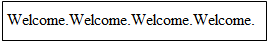## VBScript For-Next Loop

The For-Next loop can be used to execute a block of code for a specific number of times. The “VBScript For loop” specifies the counter variable and its start and end values. The Next statement increases the counter variable by one.

```For i = 1 To 5
document.write("The number is " & i & "")
Next

```

If you execute the code, you will get the output like this:## VBScript For-Step-Next Loop

By default, the counter variable is incremented by one. If you want to increase or decrease the counter variable by the value you specify, then you can use For….Step….Next loop. Suppose in the above code, you want to increment the counter by 2, then modify your code like this:

```For i = 1 To 5 Step 2
document.write("The number is " & i & "<br />")
Next
</script>
```

The output of this code will be:If you want to exit a For Next or For Step Next loop in between, then you can use Exit for the statement. Suppose you want to exit the block when the value of i becomes 3 in the above program, then you need to code like this:

```<script type="text/vbscript">
For i = 1 To 5 Step 2
If i=3 Then Exit For
document.write("The number is " & i & "<br />")
Next
</script>```

The output will be: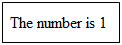## VBScript For-Each-Next Loop

If you want to repeat a block of code for each item in a collection or for each element of a VBS array, then you need to use For…Each…Next loop.

```<script type="text/vbscript">
Dim students(4)
students(0)="John"
students(1)="Hanah"
students(2)="Sarah"
students(3)="Kevin"
students(4)="Emma"

For Each x In students
document.write(x & "<br />")
Next
</script>```

The output of the above-specified code will beCode Example

```<html>

<script type="text/vbscript">
Dim name, length
length = Len(name)’Gives length of the input string

For i = 1 To length
txt = Mid(name,i,1)'Returns a specified number of characters from a string, the first parameter is the string, second parameter is the starting position and third parameter is the number of characters to return
If txt="a" or txt="A" or txt="e" or txt="E" or txt="i" or txt="I" or txt="o" or txt="O" or txt="u" or txt="U" Then
counter = counter+1
End If
Next
document.write("Hi " & name & "!!!Your name contains " & counter & " vowels.")
</script>

<body>

</body>
</html>
```

Save the file as loop.html in your preferred location. Now open the file in Internet Explorer and you will get a box asking you to enter your name. Enter a name, say Aaron, like this: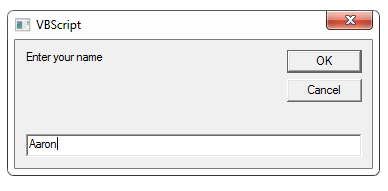Click OK and your output will look like this: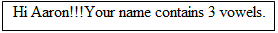## Summary

• Looping statements are used to execute the same block of code again and again.
• You will use Do-While, Do-Until and While-Wend loops when you do not know in advance how many times the block is to be executed.
• You will use For-Next, For-Next-Step and For-Each-Next loops if you already know the number of times you need to execute the block of code.

#### Top Courses